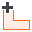## Search Results

A general polygonal fill load can be defined with the Add Fill Load option. The load magnitude can be uniform or variable, and the load application can be staged. The load magnitude is determined by multiplying the Fill Height and the Fill Unit Weight.

1. Select Loads > Fill Load2. In the Plan View, enter the vertices of the polygon as described in Entering Coordinates.
3. A load polygon must be closed. To close the polygon boundary, after all vertices are entered, right-click and select Close, or you can type c and Enter in the prompt line. This automatically and correctly closes the polygon, and saves you the trouble of having to re-enter the first vertex to complete the boundary. If the Snap option is activated, you can also close a polygon by snapping to the first vertex after all other vertices are entered.
4. After the polygon is closed, you will see the Fill Load dialog. Enter the following information:
• Load Properties (fill unit weight, fill height, staging information)
• Display Properties (optional)
5. When you are finished entering the load information, select OK. The load will be added to the model and displayed in both the Plan View and 3D View.

The parameters which define a fill load are described below.

The fill load is assumed to be Flexible and this cannot be modified.

The direction is Vertical only.

The fill load can be applied at the ground surface only. For a non-horizontal ground surface, the centroid of the load is at the ground surface.

Load magnitude is defined in terms of fill unit weight and fill height. The pressure can be uniform or variable.

Uniform Pressure

To define a uniform load magnitude, enter the Fill Unit Wt and Fill Height. This will define a load with a uniform magnitude over the area of the polygon.

Variable Pressure

To define a load magnitude which varies over the area of the polygon, select the Variable checkbox and select the Define button. You will see a dialog which allows you to define the fill height at each vertex of the polygon.

The load magnitude at each vertex is defined as a distributed load (i.e. force/area). You are NOT defining point loads, you are defining the magnitude of the distributed load, at each vertex. It is up to the user to determine the appropriate magnitude at each point, which correctly models the desired load distribution.

The load distribution over the area of the polygon is obtained by interpolation using the load magnitudes at the vertices.

If your model uses multiple stages, the load can be applied at any stage, or applied in increments at different stages using the Advanced Staging option.

Installation Stage

If you wish to apply a load at a particular stage of a multi-stage model, then use the Installation Stage option to select the desired Installation Stage.

1. Select the Advanced Staging checkbox. The dialog will expand, allowing you to enter a Load Factor and Depth for each stage.
2. The Height Factor and Weight Factor are multiplying factors for the Load Magnitude. For example:
• If you want to double the load magnitude at a given stage, enter 2 for either the Height Factor or the Weight Factor.
• If the load does not exist at a given stage, enter Height Factor and Weight Factor = 0.
• If you want the original load magnitude, enter Height Factor and Weight Factor = 1.
• If the Load Magnitude is Variable (see above), then the Load Magnitude at each vertex of the load will be multiplied by the Load Factor.

If your load is staged, it is a good idea to select the Stage Tabs, after adding the load, to check that the load is applied at the correct stage(s), and that the magnitudes and depths are correct. If not, then use the Edit Load Properties option, and make sure that the correct values have been applied at the correct stages.

## Display Properties

The appearance of a load can be customized by selecting the Display Properties button in the Define Load dialog. You will see the Load Display Properties dialog, in which you can define:

• Label - this is an optional text label/name, which can be used to identify the load, and will appear in the Info Viewer listing and Data Tips.
• Outline / Fill / Hatch - customize the colours/hatch pattern used to display the load.
• Height - the load Height option allows you to define a constant height for the load. This is for display purposes only, and has no effect on the load magnitude or analysis. If you do not define the load Height, an apparent load height is automatically determined based on the load magnitude.
Load Display Properties can be customized for each individual load. Load Display Properties can also be accessed by right-clicking on a load, and selecting Display Properties from the popup menu.